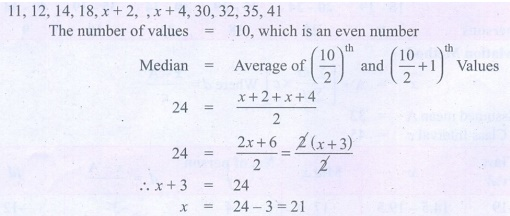Home | | Maths 9th std | Exercise 8.2: Statistics: Median-Ungrouped, Grouped Frequency Distribution

# Exercise 8.2: Statistics: Median-Ungrouped, Grouped Frequency Distribution

Maths : Statistics: Book Back, Exercise, Example Numerical Question with Answers, Solution: Exercise 8.2: Statistics: Median-Ungrouped, Grouped Frequency Distribution

Statistics: Median-Ungrouped, Grouped Frequency Distribution

Exercise 8.2

1. Find the median of the given values : 47, 53, 62, 71, 83, 21, 43, 47, 41.

Solution:2. Find the Median of the given data: 36, 44, 86, 31, 37, 44, 86, 35, 60, 51

Solution:3. The median of observation 11, 12, 14, 18, x+2, x+4, 30, 32, 35, 41 arranged in ascending order is 24. Find the values of x.

Solution:4. A researcher studying the behavior of mice has recorded the time (in seconds) taken by each mouse to locate its food by considering 13 different mice as 31, 33, 63, 33, 28, 29, 33, 27, 27, 34, 35, 28, 32. Find the median time that mice spent in searching its food.

Solution:5. The following are the marks scored by the students in the Summative Assessment examCalculate the median.

Solution:6. The mean of five positive integers is twice their median. If four of the integers are 3, 4, 6, 9 and median is 6, then find the fifth integer.

Solution:Tags : Numerical Problems with Answers, Solution | Statistics | Maths , 9th Maths : UNIT 8 : Statistics
Study Material, Lecturing Notes, Assignment, Reference, Wiki description explanation, brief detail
9th Maths : UNIT 8 : Statistics : Exercise 8.2: Statistics: Median-Ungrouped, Grouped Frequency Distribution | Numerical Problems with Answers, Solution | Statistics | Maths